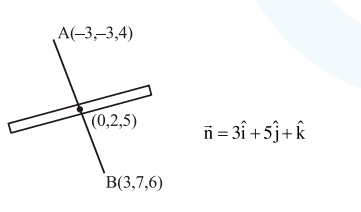# The plane which bisects the line segment joining the points`
Question:

The plane which bisects the line segment joining the points $(-3,-3,4)$ and $(3,7,6)$ at right angles, passes through which one of the following points ?

1. $(4,-1,7)$

2. $(4,1,-2)$

3. $(-2,3,5)$

4. $(2,1,3)$

Correct Option: , 2

Solution:$p: 3(x-0)+5(y-2)+1(z-5)=0$

$3 x+5 y+z=15$

$\therefore$ Option (2)